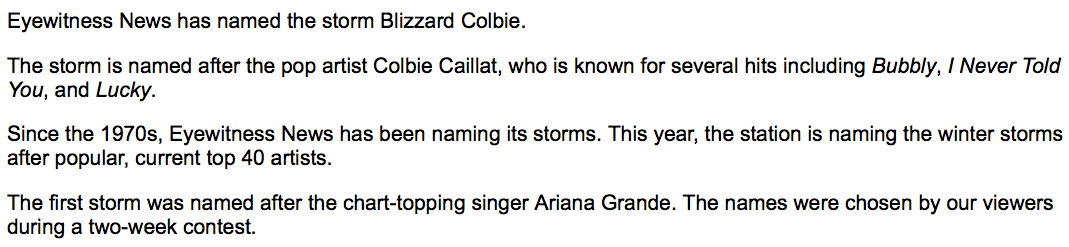## Sunday, January 25, 2015

### Storm 'Colbie' Caillat, the East Coast Blizzard

Meteorologist Mike "Michael" Cameron?  I wonder if he was born to be?
• Mike = 4+9+2+5 = 20
• Cameron = 3+1+4+5+9+6+5 = 33
• Michael = 4+9+3+8+1+5+3 = 33Please notice that this blizzard is being given a name, 'Colbie', based on the pop artist Colbie Caillat.
• Colbie = 3+15+12+2+9+5 = 46
• Caillat = 3+1+9+12+12+1+20 = 58
• CC = 33
Colbie Caillat was born May 28, 1985.
• 5/28 = 5+28 = 33
• 5/28/85 = 5+28+85 = 118
• 5/28/1985 = 5+2+8+1+9+8+5 = 38
Since when are storms named after Pop Stars?  They named the last Blizzard after Ariana Grande, who was born June 26, 1993.
• Ariana = 1+18+9+1+14+1 = 44
• Grande = 7+18+1+14+4+5 = 49
• Ariana Grande = 93
Ariana Grande's life numbers are as follows.
• 6/26 = 6+26 = 32
• 6/26/93 = 6+26+93 = 125
• 6/26/1993 = 6+2+6+1+9+9+3 = 36